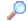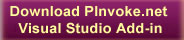SearchDirectory

Desktop Functions:

Smart Device Functions:GlossaryShow Recent ChangesSubscribe (RSS)Misc. Pages
EMR_ (Constants)

Summary
The iType field of the Enhanced Meta File structures is one of the following

#### C# Constants:

// Constants for Enhanced Metafile records
const int
EMR_POLYBEZIER         = 2,
EMR_POLYGON            = 3,
EMR_POLYLINE           = 4,
EMR_POLYBEZIERTO           = 5,
EMR_POLYLINETO         = 6,
EMR_POLYPOLYLINE           = 7,
EMR_POLYPOLYGON        = 8,
EMR_SETWINDOWEXTEX         = 9,
EMR_SETWINDOWORGEX         = 10,
EMR_SETVIEWPORTEXTEX       = 11,
EMR_SETVIEWPORTORGEX       = 12,
EMR_SETBRUSHORGEX          = 13,
EMR_EOF            = 14,
EMR_SETPIXELV          = 15,
EMR_SETMAPPERFLAGS         = 16,
EMR_SETMAPMODE         = 17,
EMR_SETBKMODE          = 18,
EMR_SETPOLYFILLMODE        = 19,
EMR_SETROP2            = 20,
EMR_SETSTRETCHBLTMODE      = 21,
EMR_SETTEXTALIGN           = 22,
EMR_SETTEXTCOLOR           = 24,
EMR_SETBKCOLOR         = 25,
EMR_OFFSETCLIPRGN          = 26,
EMR_MOVETOEX           = 27,
EMR_SETMETARGN         = 28,
EMR_EXCLUDECLIPRECT        = 29,
EMR_INTERSECTCLIPRECT      = 30,
EMR_SCALEVIEWPORTEXTEX     = 31,
EMR_SCALEWINDOWEXTEX       = 32,
EMR_SAVEDC             = 33,
EMR_RESTOREDC          = 34,
EMR_SETWORLDTRANSFORM      = 35,
EMR_MODIFYWORLDTRANSFORM       = 36,
EMR_SELECTOBJECT           = 37,
EMR_CREATEPEN          = 38,
EMR_CREATEBRUSHINDIRECT    = 39,
EMR_DELETEOBJECT           = 40,
EMR_ANGLEARC           = 41,
EMR_ELLIPSE            = 42,
EMR_RECTANGLE          = 43,
EMR_ROUNDRECT          = 44,
EMR_ARC            = 45,
EMR_CHORD              = 46,
EMR_PIE            = 47,
EMR_SELECTPALETTE          = 48,
EMR_CREATEPALETTE          = 49,
EMR_SETPALETTEENTRIES      = 50,
EMR_RESIZEPALETTE          = 51,
EMR_REALIZEPALETTE         = 52,
EMR_EXTFLOODFILL           = 53,
EMR_LINETO             = 54,
EMR_ARCTO              = 55,
EMR_POLYDRAW           = 56,
EMR_SETARCDIRECTION        = 57,
EMR_SETMITERLIMIT          = 58,
EMR_BEGINPATH          = 59,
EMR_ENDPATH            = 60,
EMR_CLOSEFIGURE        = 61,
EMR_FILLPATH           = 62,
EMR_STROKEANDFILLPATH      = 63,
EMR_STROKEPATH         = 64,
EMR_FLATTENPATH        = 65,
EMR_WIDENPATH          = 66,
EMR_SELECTCLIPPATH         = 67,
EMR_ABORTPATH          = 68,
EMR_GDICOMMENT         = 70,
EMR_FILLRGN            = 71,
EMR_FRAMERGN           = 72,
EMR_INVERTRGN          = 73,
EMR_PAINTRGN           = 74,
EMR_EXTSELECTCLIPRGN       = 75,
EMR_BITBLT             = 76,
EMR_STRETCHBLT         = 77,
EMR_PLGBLT             = 79,
EMR_SETDIBITSTODEVICE      = 80,
EMR_STRETCHDIBITS          = 81,
EMR_EXTCREATEFONTINDIRECTW     = 82,
EMR_EXTTEXTOUTA        = 83,
EMR_EXTTEXTOUTW        = 84,
EMR_POLYBEZIER16           = 85,
EMR_POLYGON16          = 86,
EMR_POLYLINE16         = 87,
EMR_POLYBEZIERTO16         = 88,
EMR_POLYLINETO16           = 89,
EMR_POLYPOLYLINE16         = 90,
EMR_POLYPOLYGON16          = 91,
EMR_POLYDRAW16         = 92,
EMR_CREATEMONOBRUSH        = 93,
EMR_CREATEDIBPATTERNBRUSHPT    = 94,
EMR_EXTCREATEPEN           = 95,
EMR_POLYTEXTOUTA           = 96,
EMR_POLYTEXTOUTW           = 97,
EMR_SETICMMODE         = 98,
EMR_CREATECOLORSPACE       = 99,
EMR_SETCOLORSPACE          =100,
EMR_DELETECOLORSPACE       =101,
EMR_GLSRECORD          =102,
EMR_GLSBOUNDEDRECORD       =103,
EMR_PIXELFORMAT        =104,
EMR_RESERVED_105           =105,
EMR_RESERVED_106           =106,
EMR_RESERVED_107           =107,
EMR_RESERVED_108           =108,
EMR_RESERVED_109           =109,
EMR_RESERVED_110           =110,
EMR_COLORCORRECTPALETTE    =111,
EMR_SETICMPROFILEA         =112,
EMR_SETICMPROFILEW         =113,
EMR_ALPHABLEND         =114,
EMR_SETLAYOUT          =115,
EMR_TRANSPARENTBLT         =116,
EMR_RESERVED_117           =117,
EMR_RESERVED_119           =119,
EMR_RESERVED_120           =120,
EMR_COLORMATCHTOTARGETW    =121,
EMR_CREATECOLORSPACEW      =122;

TODO

#### Notes:

None.

Do you have...

• corrections to the existing content?
• additional languages you want to include?

Select "Edit This Page" on the right hand toolbar and edit it!Access PInvoke.net directly from VS:Terms of UseEdit This PageFind ReferencesShow Printable VersionRevisions Show changes